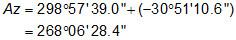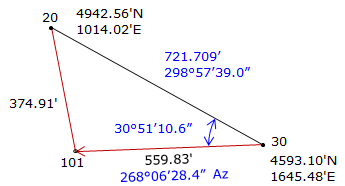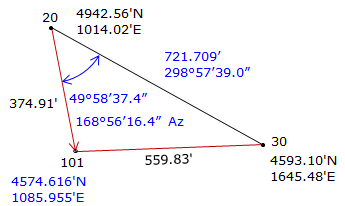### Article Index

Two different intersection types are solved using triangle- and arc-based methods to demonstrate the different computation process. Additional decimal places will be carried in computations to minimize rounding errors.

### 1. Distance-distanceGiven the information on the diagram, determine the coordinates of point 101. Figure E-1 Distance-distance example

Step (1) For both methods is to inverse along the base line 30-20#### a. Triangle-based method

 Step (2) Compute angle at 30 by Law of Cosines.Step (3) Compute direction from 30 to 101.Step (4) Perform a forward computation from 30 to 101.Math Check: Compute coordinates from 20.

 Step (1) Compute angle at 20 by Law of Sines.Step (2) Compute direction from 20 to 101.Step (3) Perform a forward computation from 20 to 101.Both coordinates check.#### b. Arc-based method

Step (2) Set up and solve Equations D-6 through D-9.Step (3) Use Equations D-10 and D-11 to compute the two intersection pointsStep (4) Of the two, select the appropriate intersection point.

Point 101 is located southwest of the base line.

 Point North East From base line 1011 5077.015 1363.991 north east 1012 4574.617 1085.956 south west

The correct intersection point is 1012: (4754.617 ft N, 1085.956 ft E), same as the triangle-based solution.